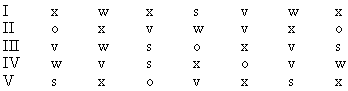Please Support the Monkey! Tell All your Friends and Teachers

PRACTICE TEST 4

Section VI: Analytical Section

Time: 30 Minutes                                      Questions 25

 Directions: All questions are based on a passage or set of conditions. While answering a few of the questions, you would find it useful to draw a rough diagram. To answer any question choose the answer you think is most appropriate among the given options. .

Questions 1 - 5

(1) All the rows contains at least two letters, which occur only once in the row.

(2) In every row the last three letters are different.

(3) In every row of letters, the first two letters and the last two letters are the same.

(4) All the rows contain more than four different letters.1. Which of the above rows fulfills all the conditions mentioned?

(A) Row IV and V only
(B) Row IV only
(C) Rows I and II only
(D) Row I only
(E) Row III only

2. Which rows among the above do not satisfy the fourth condition?

(A) Rows II and III only
(B) Rows I and V only
(C) Row II only
(D) Rows I, II and V only
(E) Row V only

3. Which letter if filled in the blank will satisfy all the conditions?

o         w      s        v       .....      w       o

(A) o
(B) v
(C) x
(D) s
(E) w

Test 4

Section 1 : Verbal Section

Section 2 : Quantitative Section

Section 3 : Analytical Section

Section 4 : Quantitative Section

Section 5 : Verbal Section

Section 6 : Analytical Section

Section 7 : Analytical Section

Answer Key To Test 4

Answer Explanation To Test 4
Section 1 : Verbal Section

Section 2 : Quantitative Section

Section 3 : Analytical Section
Section 4 : Quantitative Section
Section 5 : Verbal Section

Section 6 : Analytical Section
Section 7 : Analytical Section